﻿ 基于星间链路体制的北斗卫星时间同步可行性分析文章快速检索 高级检索
 大地测量与地球动力学2018, Vol. 38Issue (12): 1274-1279  DOI: 10.14075/j.jgg.2018.12.012### 引用本文WEN Xufeng, HAO Jinming, HU Xiaogong, et al. Feasibility Analysis of Time Synchronization of Beidou Satellite Using Inter-Satellite Observation Data[J]. Journal of Geodesy and Geodynamics, 2018, 38(12): 1274-1279.### Foundation support

National Natural Science Foundation of China, No. 61603397; Open Fund of State Key Laboratory of Geodesy and Earth's Dynamics, No. SKLGED2017-3-3-E.

### About the first author

WEN Xufeng, postgraduate, majors in autonomous navigation of navigation constellation, E-mail: wenxufeng201@126.com.

### 文章历史

1. 信息工程大学地理空间信息学院，郑州市科学大道62号，450001;
2. 中国科学院上海天文台，上海市南丹路80号，200030;
3. 北京卫星导航中心，北京市北清路22号，100094;
4. 大地测量与地球动力学国家重点实验室，武汉市徐东大街340号，430077

1 星地双向时间同步数学模型

 $\begin{array}{l} {\rho _{{\rm{AB}}}}({t_1}) = \left| {\overrightarrow {{r_{\rm{B}}}} ({t_1}) - \overrightarrow {{r_{\rm{A}}}} ({t_1} - \Delta {t_1})} \right| + \\ \;\;\;\;\;\;c\left( {{\tau _{\rm{B}}}({t_1}) - {\tau _{\rm{A}}}({t_1})} \right) + {\delta _{{\rm{AB}}}}\\ {\rho _{{\rm{BA}}}}({t_2}) = \left| {\overrightarrow {{r_{\rm{A}}}} ({t_2}) - \overrightarrow {{r_{\rm{B}}}} ({t_2} - \Delta {t_2})} \right| + \\ \;\;\;\;\;\;c\left( {{\tau _{\rm{A}}}({t_2}) - {\tau _{\rm{B}}}({t_2})} \right) + {\delta _{{\rm{BA}}}} \end{array}$ (1)

 $\begin{array}{l} {\delta _{{\rm{AB}}}} = c \cdot \\ \left( {\tau _{\rm{A}}^{{\rm{Send}}} + \tau _{\rm{B}}^{{\rm{Rcv}}} + \tau _{\rm{B}}^{{\rm{ant}}} + {\tau _{{\rm{rel}}}} + {\tau _{{\rm{ion}}}} + {\tau _{{\rm{trop}}}} + \tau _{{\rm{sagnac}}}^{{\rm{AB}}}} \right)\\ {\delta _{{\rm{BA}}}} = c \cdot \\ \left( {\tau _{\rm{B}}^{{\rm{Send}}} + \tau _{\rm{A}}^{{\rm{Rcv}}} + \tau _{\rm{A}}^{{\rm{ant}}} + {\tau _{{\rm{rel}}}} + {\tau _{{\rm{ion}}}} + {\tau _{{\rm{trop}}}} + \tau _{{\rm{sagnac}}}^{{\rm{BA}}}} \right) \end{array}$ (2)

BD-2应用L波段星地双向时频传递技术(TWSTFT)获取卫星钟相对于BDT的偏差，该体制中卫星和地面站在同一钟面时发射、接收伪距信号[3, 8]，则式(1)中t1=t2。而ISL时分体制双向伪距观测量需要归算到同一钟面才能进行钟差解算，设定将两个观测量归算到t0时刻：

 $\begin{array}{l} {\rho _{{\rm{AB}}}}({t_0}) = {\rho _{{\rm{AB}}}}({t_1}) + {\rm{d}}{\rho _{{\rm{AB}}}} = \\ \left| {\overrightarrow {{r_{\rm{B}}}} ({t_0}) - \overrightarrow {{r_{\rm{A}}}} ({t_0})} \right| + c\left( {{\tau _{\rm{B}}}({t_0}) - {\tau _{\rm{A}}}({t_0})} \right) + {\delta _{{\rm{AB}}}}\\ {\rho _{{\rm{BA}}}}({t_0}) = {\rho _{{\rm{BA}}}}({t_2}) + {\rm{d}}{\rho _{{\rm{BA}}}} = \\ \left| {\overrightarrow {{r_{\rm{A}}}} ({t_0}) - \overrightarrow {{r_{\rm{B}}}} ({t_0})} \right| + c\left( {{\tau _{\rm{A}}}({t_0}) - {\tau _{\rm{B}}}({t_0})} \right) + {\delta _{{\rm{BA}}}} \end{array}$ (3)

ρAB(t0)和ρSA(t0)作差，可将观测量的测距信息和钟差信息解耦，获得相对钟差信息：

 $\begin{array}{l} \;\;\;\;\;\;\;\;\;{\tau _{\rm{A}}}({t_0}) - {\tau _{\rm{B}}}({t_0}) = \\ \frac{{{\rho _{{\rm{AB}}}}({t_0}) - {\rho _{{\rm{BA}}}}({t_0})}}{{2c}} - \frac{{{\delta _{{\rm{AB}}}} - {\delta _{{\rm{BA}}}}}}{{2c}} \end{array}$ (4)

2 星地钟差观测量分析

2.1 7 d拟合结果分析表 1 星地钟差7 d拟合参数比较 Tab. 1 Comparison of 7 days' fitting parameters of satellite-ground clock offset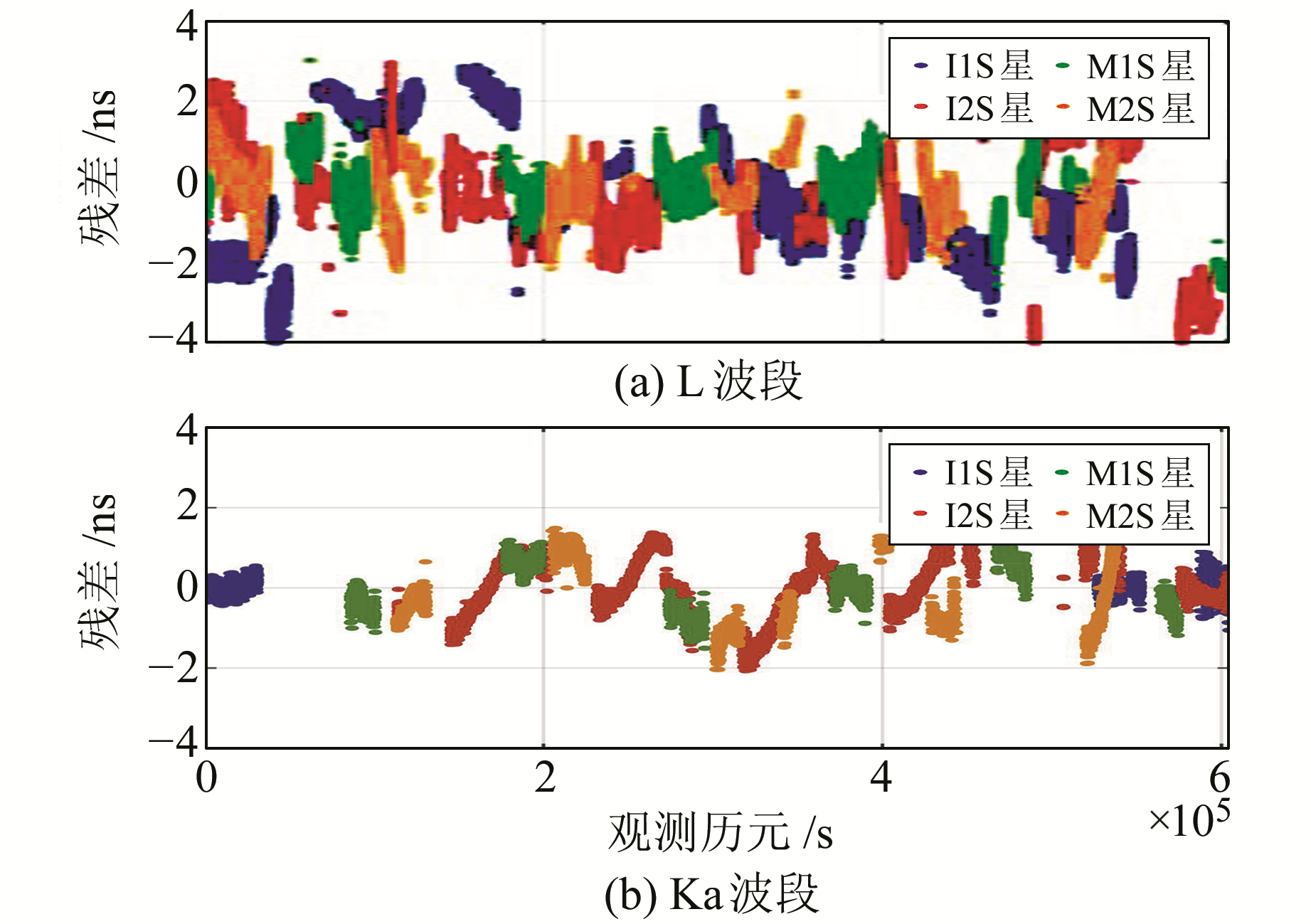图 1 星地钟差7 d拟合残差 Fig. 1 7 days' fitting residuals of satellite-ground clock offset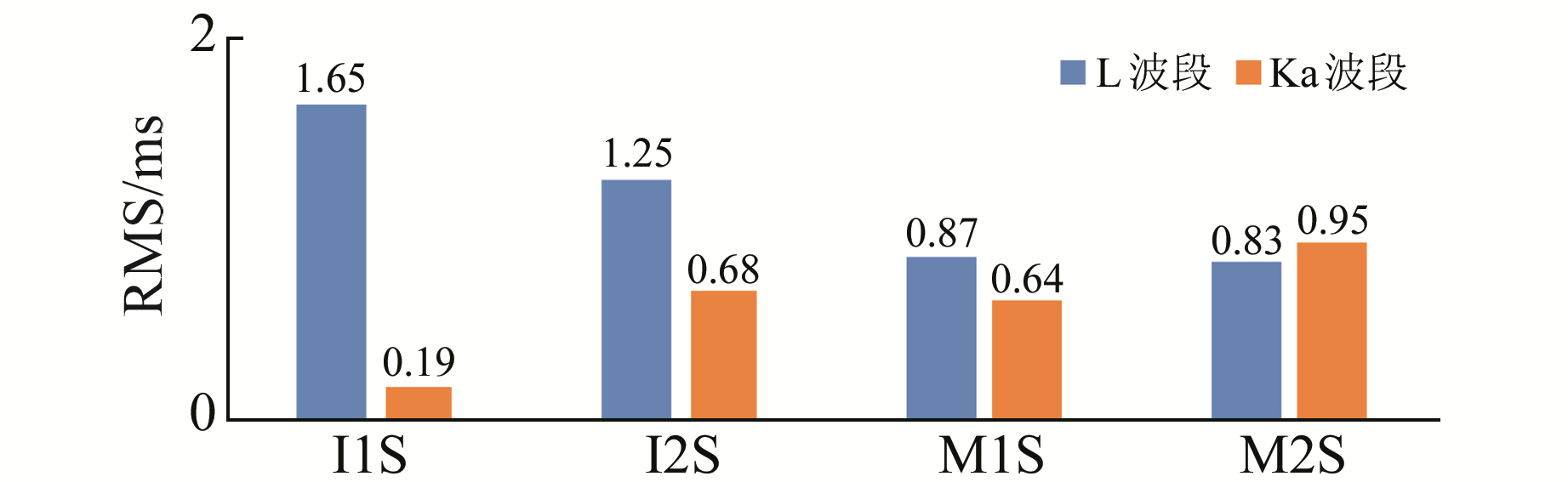图 2 星地钟差7天拟合残差RMS比较 Fig. 2 RMS comparison of 7 days' fitting residuals of satellite-ground clock offset
2.2 3 d拟合结果分析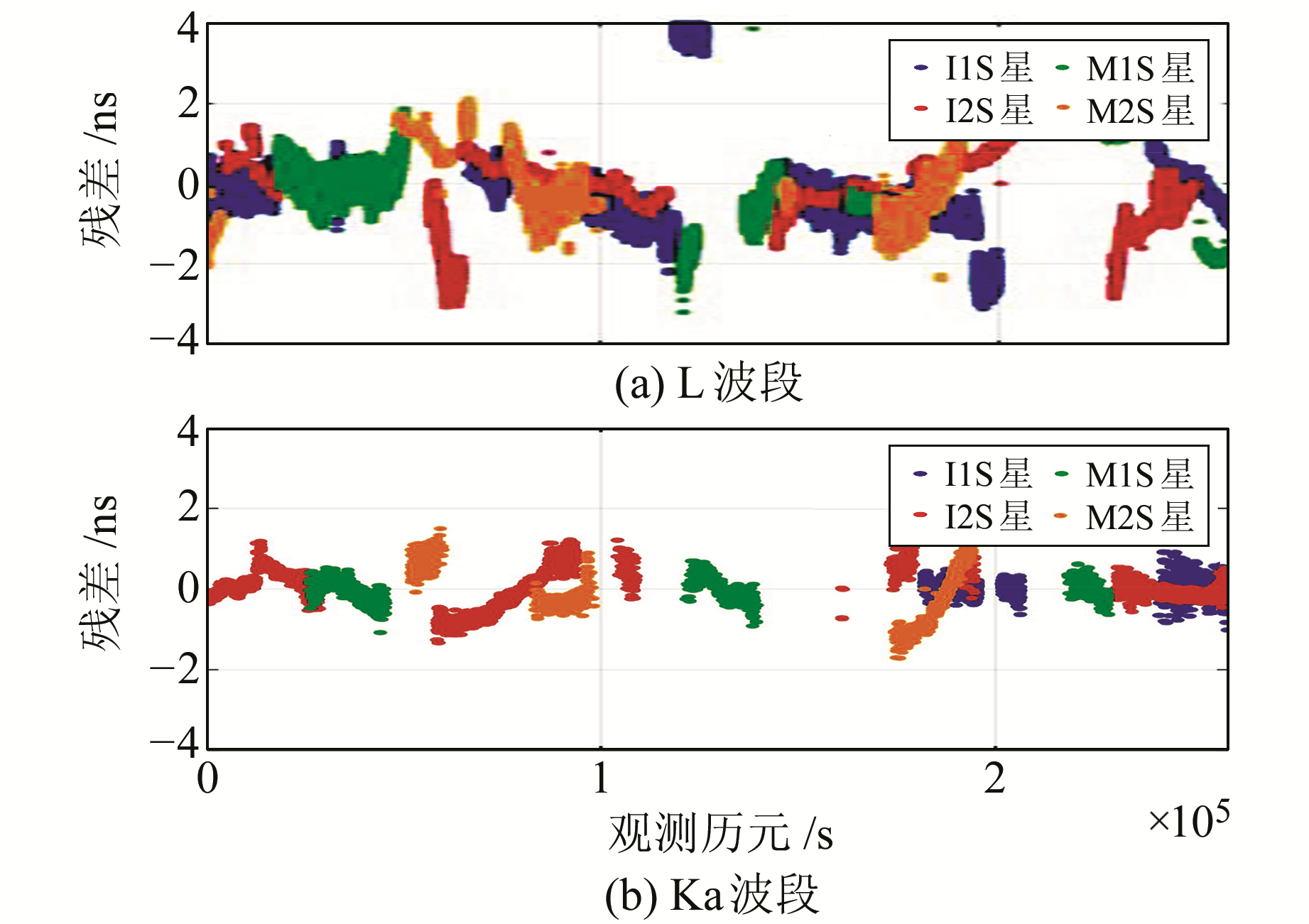图 3 星地钟差3 d拟合残差 Fig. 3 3 days' fitting residuals of satellite-ground clock offset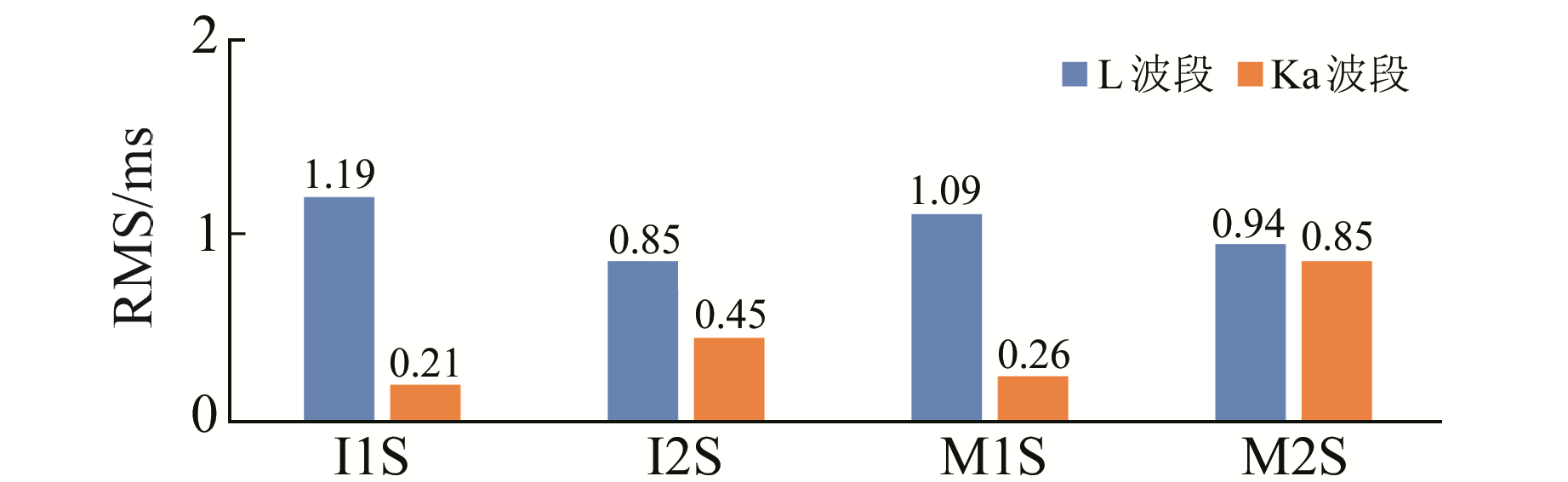图 4 星地钟差3 d拟合残差RMS比较 Fig. 4 RMS comparison of 3 days' fitting residuals of satellite-ground clock offset
2.3 1 d拟合结果分析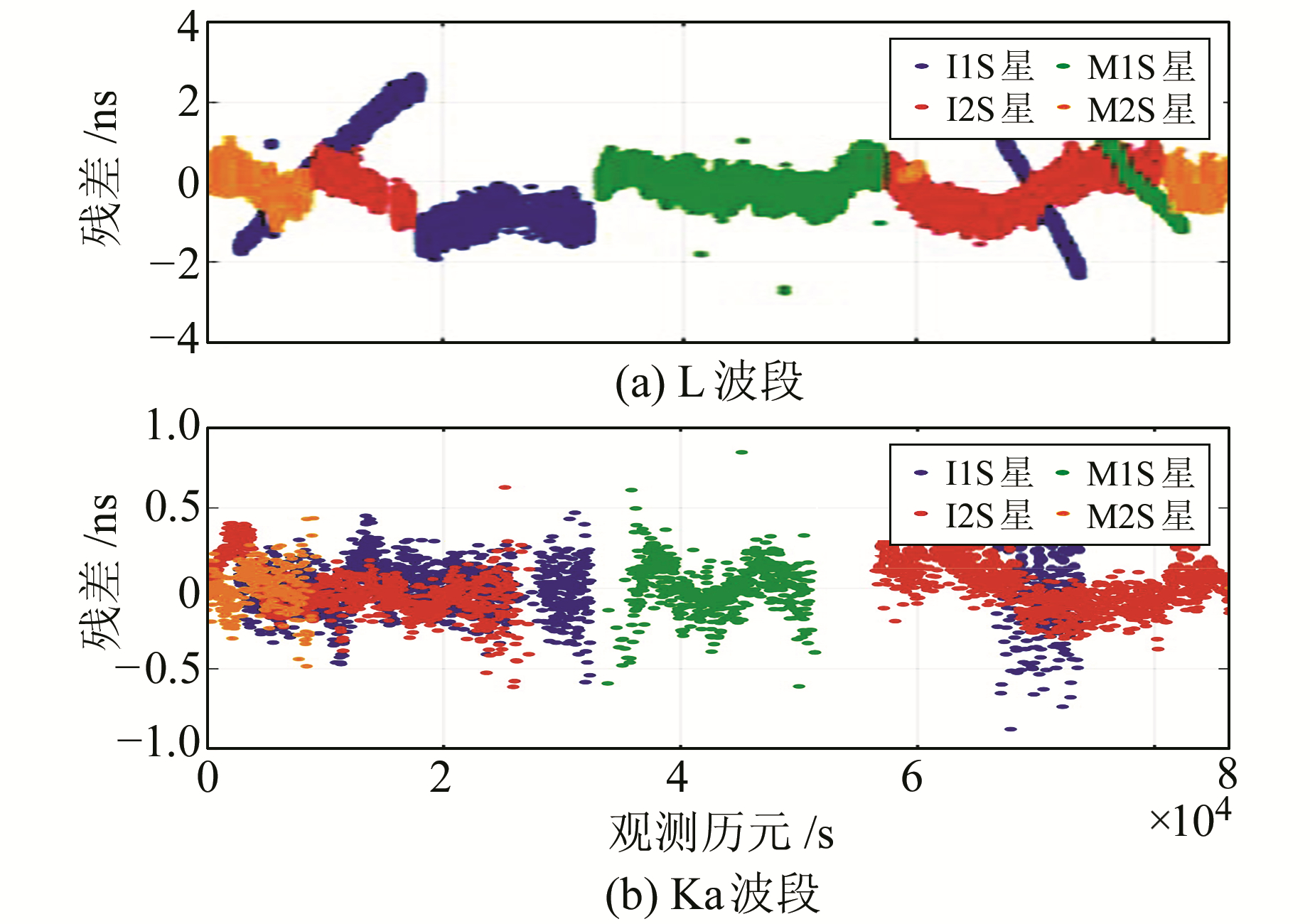图 5 星地钟差1 d拟合残差 Fig. 5 1 day's fitting residuals of satellite-ground clock offset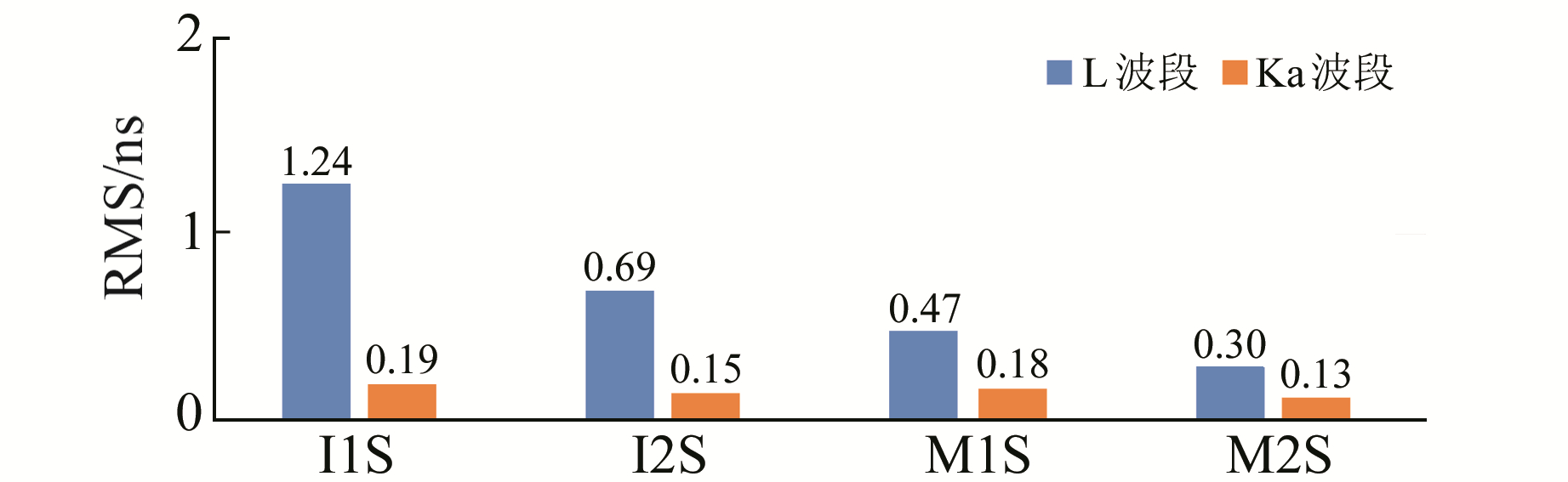图 6 星地钟差1 d拟合残差RMS比较 Fig. 6 RMS comparison of 1 day's fitting residuals of satellite-ground clock offset
2.4 1 h拟合结果分析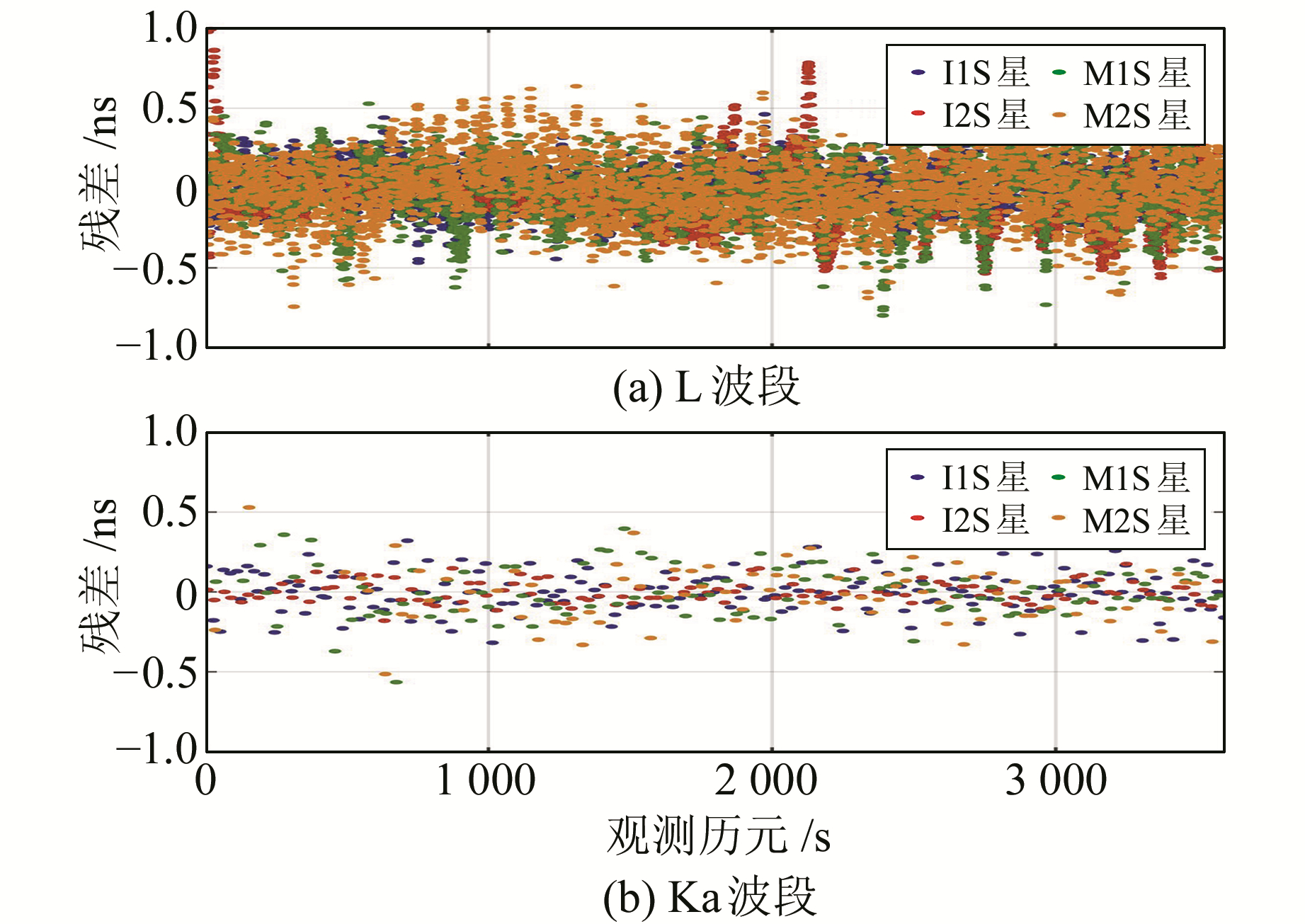图 7 星地钟差1 h拟合残差 Fig. 7 1 hour's fitting residuals of satellite-ground clock offset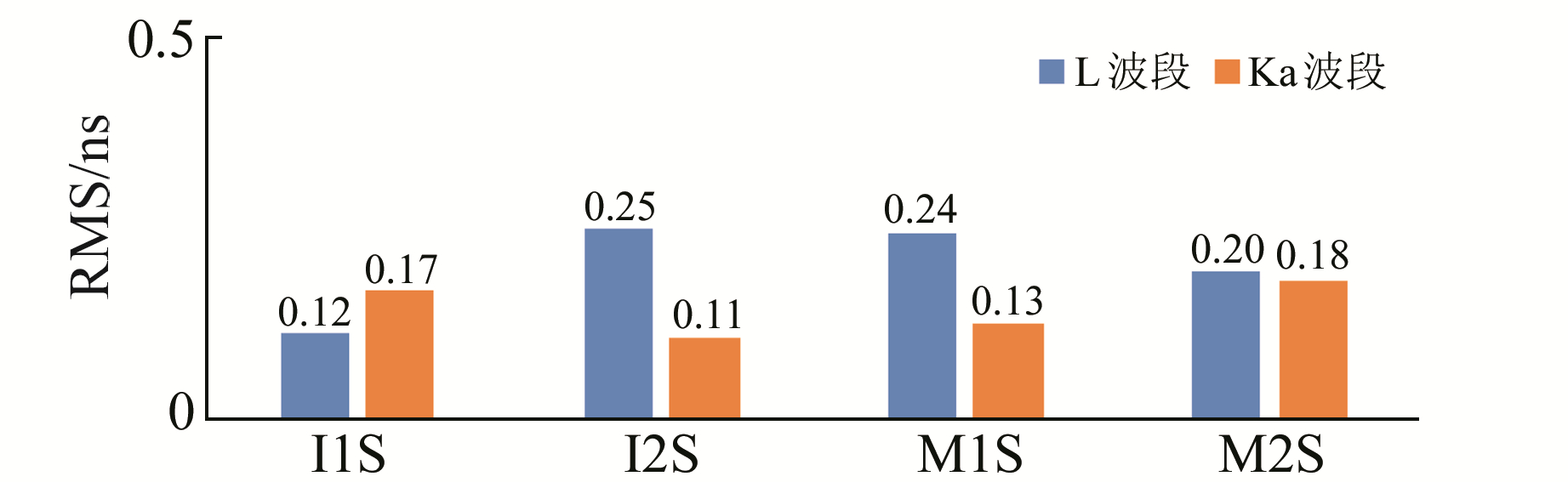图 8 星地钟差1 h拟合残差RMS比较 Fig. 8 RMS comparison of 1 hour's fitting residuals of satellite-ground clock offset
3 钟差预报精度分析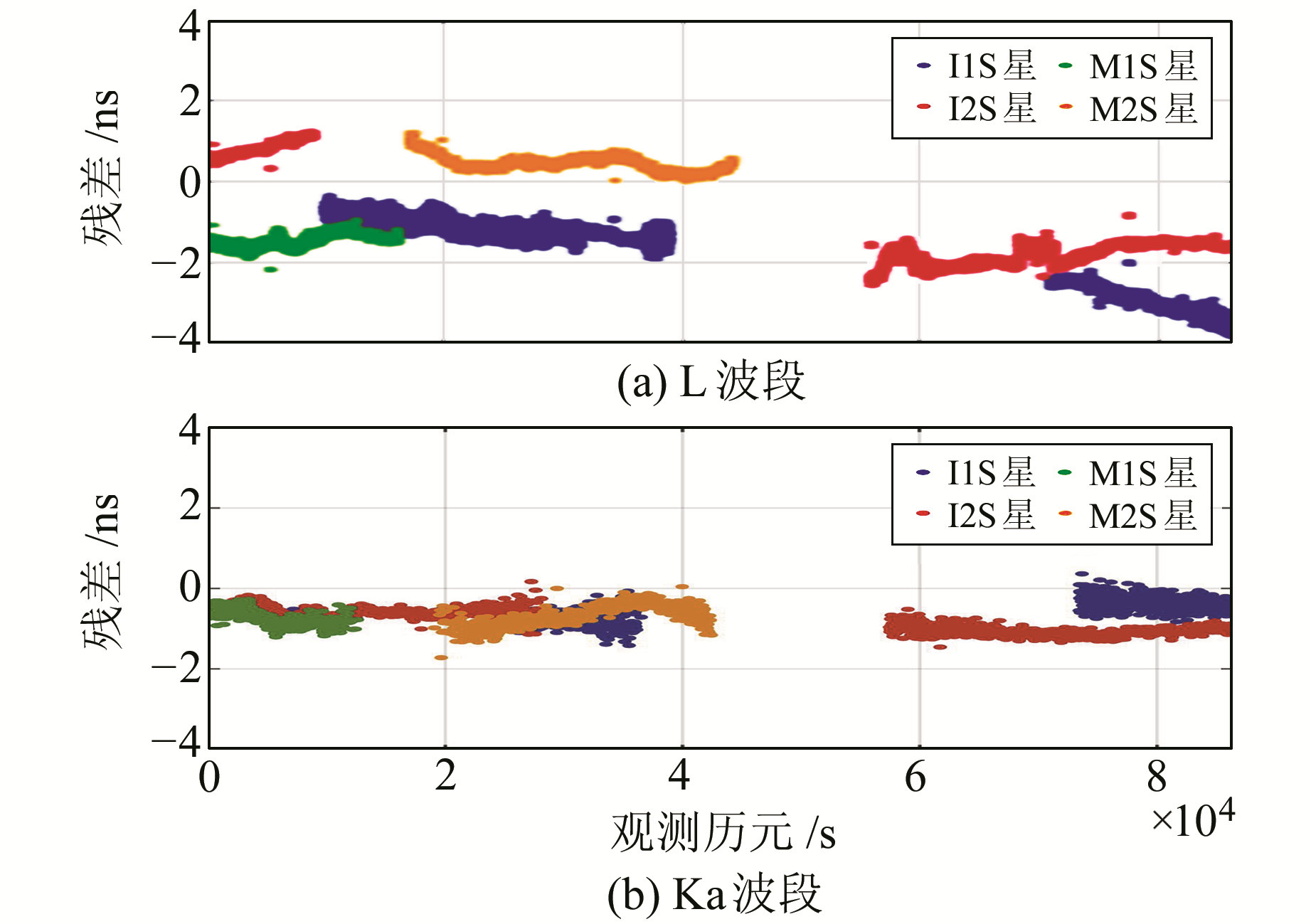图 9 星地钟差预报误差 Fig. 9 Prediction errors of satellite-ground clock offset

4 星地星间联合钟差观测

 $\Delta {t'_{{\rm{M1S - BDT}}}} = \Delta {t_{{\rm{M1S - I1S}}}} + \Delta {t_{{\rm{I1S - BDT}}}}$ (5)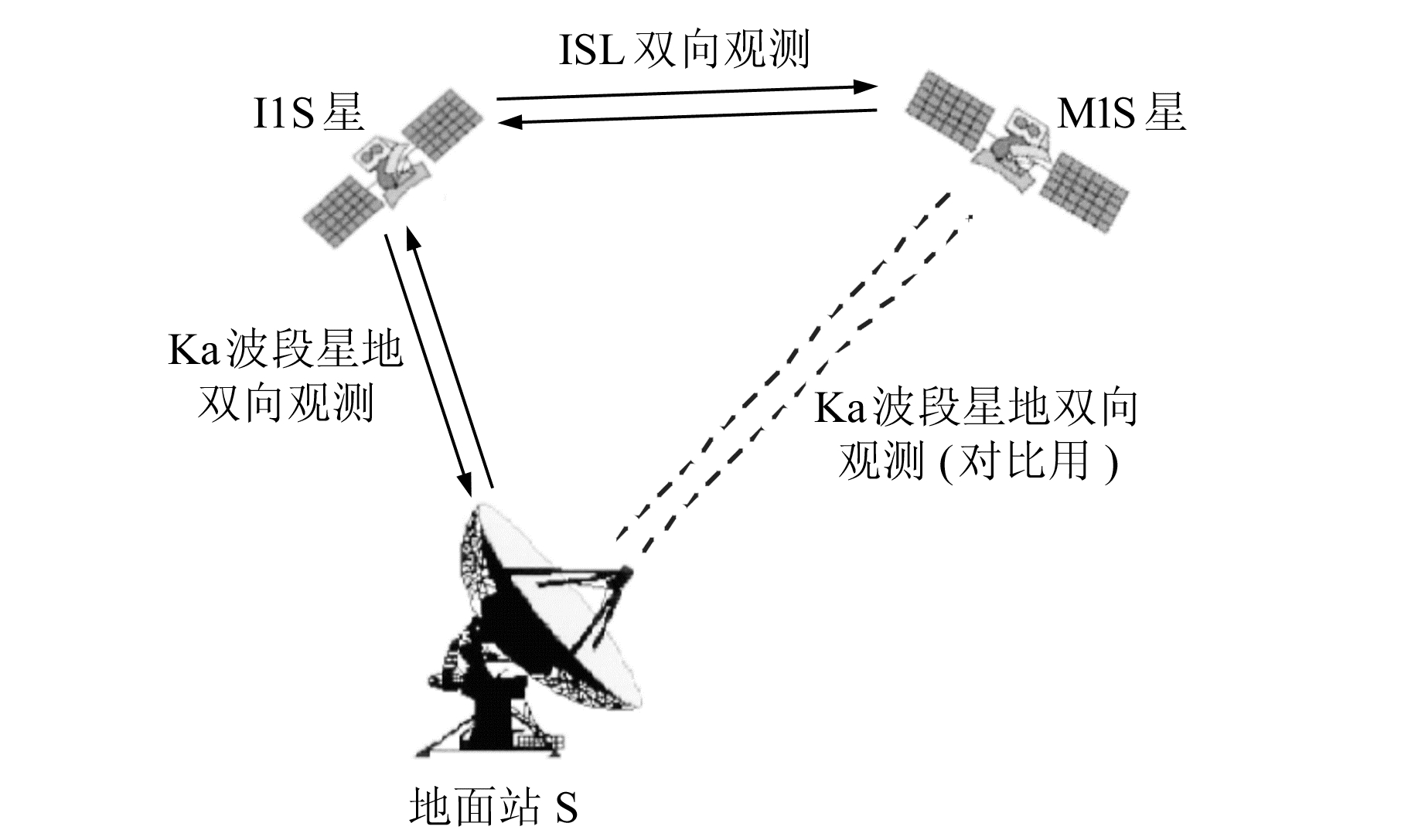图 10 星地星间测量示意图 Fig. 10 A schematic diagram of inter-satellite and satellite-ground measurement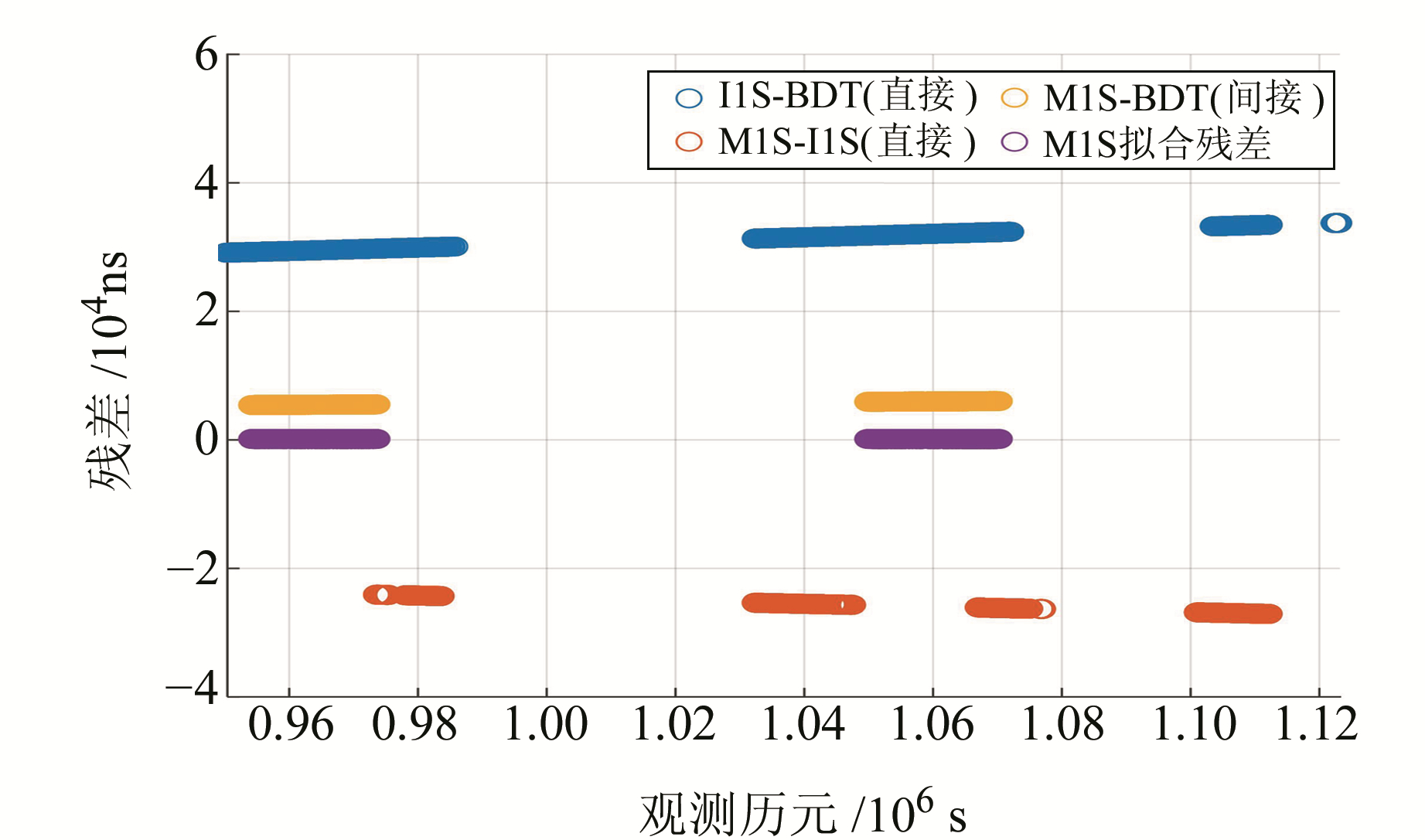图 11 星地联合钟差解算及拟合残差 Fig. 11 Fitting residuals of satellite-ground joint clock calculation

5 结语

1) ISL体制钟差拟合参数与L波段一致性较好，其7 d、3 d、1 d和1 h弧段的星地时间同步误差分别为1 ns、0.85 ns、0.2 ns和0.2 ns。其中，1 d弧段拟合精度比L波段有明显提高，且在1 d弧段上ISL体制的观测量已经不存在明显的系统差，再缩小拟合时长对精度提高不明显，故1 d为最优拟合时长。

2) ISL钟差1 d预报精度为2 ns，略好于L波段，钟差预报较为可靠。

3) ISL星间观测可靠，2 d间接钟差观测拟合残差为0.52 ns。

  Ananda M P, Bernstein H, Cunningham K E, et al. Global Positioning System(GPS) Autonomous Navigation[J]. IEEE Position Location & Navigation Symposium, 2002, 27(1): 497-508 (0)  Rajan J A, Brodie P, Rawicz H. Modernizing GPS Autonomous Navigation with Anchor Capability[C]. International Technical Meeting of the Satellite Division of the Institute of Navigation, 2003 (0)  Liu L, Zhu L F, Han C H, et al. The Model of Radio Two-Way Time Comparison between Satellite and Station and Experimental Analysis[J]. Chinese Astronomy & Astrophysics, 2009, 33(4): 431-439 (0)  李瑞锋, 王元明. 星地双向时间同步技术在COMPASS中的应用[J]. 武汉大学学报:信息科学版, 2013, 38(7): 842-844 (Li Ruifeng, Wang Yuanming. Application of Two-Way Satellite-Ground Time Synchronization in COMPASS[J]. Geomatics and Information Science of Wuhan University, 2013, 38(7): 842-844) (0)  唐成盼, 胡小工, 周善石, 等. 利用星间双向测距数据进行北斗卫星集中式自主定轨的初步结果分析[J]. 中国科学:物理学力学天文学, 2017, 47(2): 95-105 (Tang Chengpan, Hu Xiaogong, Zhou Shanshi, et al. Centralized Autonomous Orbit Determination of Beidou Navigation Satellites with Inter-Satellite Link Measurements: Preliminary Results[J]. Scientia Sinica: Physica, Mechanica & Astronomica, 2017, 47(2): 95-105) (0)  吕宏春, 卢晓春, 武建锋. 星间链路体制下的星地双向时间同步方法[J]. 宇航学报, 2017, 38(7): 728-734 (Lǖ Hongchun, Lu Xiaochun, Wu Jianfeng. A Method of Two Way Satellite Ground Time Synchronization Under Inter Satellite Links System[J]. Journal of Astronautics, 2017, 38(7): 728-734) (0)  潘军洋, 胡小工, 唐成盼, 等. 北斗新一代卫星时分体制星间链路测量的系统误差标定[J]. 科学通报, 2017, 62(23): 2 671-2 679 (Pan Junyang, Hu Xiaogong, Tang Chengpan, et al. System Error Calibration for Time Division Multiple Address Inter-Satellite Equipment of Next-Generation Beidou Satellites[J]. Chinese Science Bulletin, 2017, 62(23): 2 671-2 679) (0)  Han C, Cai Z, Lin Y, et al. Time Synchronization and Performance of Beidou Satellite Clocks in Orbit[J]. International Journal of Navigation and Observation, 2013, Article ID: 371450 (0)  方琳.基于双向星间链路的自主时间同步仿真分析[D].北京: 中国科学院研究生院(国家授时中心), 2013 (Fang Lin. Simulation and Analysis of Autonomous Time Synchronization Based on Two-Way Inter-Satellite Link[D]. Beijing: University of Chinese Academy of Science(National Time Service Center), 2013) (0)
Feasibility Analysis of Time Synchronization of Beidou Satellite Using Inter-Satellite Observation Data
WEN Xufeng1HAO Jinming1     HU Xiaogong2     TANG Chengpan2     WANG Dongxia3,4     CHEN Liucheng3     CHANG Zhiqiao3     ZHU Lingfeng3     WU Shan3     LI Xiaojie3     JIAO Bo1
1. School of Surveying and Mapping, Information Engineering University, 62 Kexue Road, Zhengzhou 450001, China;
2. Shanghai Astronomical Observatory, CAS, 80 Nandan Road, Shanghai 200030, China;
3. Beijing Satellite Navigation Center, 22 Beiqing Road, Beijing 100094, China;
4. State Key Laboratory of Geodesy and Earth's Dynamics, 340 Xudong Street, Wuhan 430077, China
Abstract: Beidou-3 experimental satellites are equipped with Ka band inter-satellite link(ISL) equipment, which provides satellite-ground orbit determination and time synchronization observation means of ISL mode. The mathematical model of synchronous observation of time-division satellite-ground two-way ISL mode is derived. Taking the satellite-ground data of ISL as a sample, the feasibility of time synchronization in ISL system is analyzed and compared with L band observations. The results show that the fitting parameters of clock offset in ISL system are consistent with those of L band. The fitting accuracies within 7 days, 3 days, 1 day and 1 hour are respectively 1 ns, 0.85 ns, 0.2 ns and 0.2 ns, which, especially for 1 day fitting, are better than those of L band. At the same time, the clock offset prediction accuracy of the next day is analyzed by using seven-day measurements of the satellite ground clock offset. The prediction error of ISL satellite clock offset is within 2 ns. Finally, the satellite-ground and inter-satellites combined observation is carried out. The error in 2 days observation is 0.52 ns. The feasibility of ISL observation is verified.
Key words: Beidou-3 new generation experimental satellites; inter-satellite link; satellite-ground time synchronous; clock offset prediction